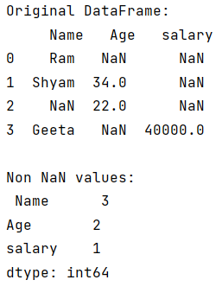# Count number of non-NaN entries in every column of Dataframe

Given a Pandas DataFrame, we have to count number of non-NaN entries in every column.
Submitted by Pranit Sharma, on July 07, 2022

Pandas is a special tool that allows us to perform complex manipulations of data effectively and efficiently. Inside pandas, we mostly deal with a dataset in the form of DataFrame. DataFrames are 2-dimensional data structures in pandas. DataFrames consist of rows, columns, and data.

## Problem statement

While creating a DataFrame or importing a CSV file, there could be some NaN values in the cells. NaN values mean "Not a Number" which generally means that there are some missing values in the cell. To deal with this type of data, you can either remove the particular row (if the number of missing values is low) or you can handle these values. For handling these values, you might need to count the number of NaN values or you need to count the number of non-NaN values.

## Count number of non-NaN entries in every column

To count number of non-NaN entries in every column of Dataframe, we will use pandas.DataFrame.count() Method which will count the non-NaN values in each column.

Consider the below-given syntax:

```DataFrame.count(
axis=0,
level=None,
numeric_only=False
)
```

Let us understand with the help of an example,

## Python program to count number of non-NaN entries in every column of Dataframe

```# Importing pandas package
import pandas as pd

# Importing numpy package
import numpy as np

# Creating a dictionary
d = {
'Name':["Ram","Shyam",np.NaN,"Geeta"],
'Age':[np.NaN,34,22,np.NaN],
"salary":[np.NaN,np.NaN,np.NaN,40000]}

# Creating a DataFrame
df = pd.DataFrame(d)

# Display original DataFrame
print("Original DataFrame:\n",df,"\n")

# Counting non NaN values
print("Non NaN values:\n",df.count())
```

## Output

The output of the above program will be: